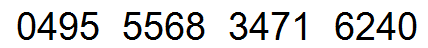# 6050 Matrices From Tables & Numbers

8 Questions | Total Attempts: 359Settings• 1.
Above is a credit card number. If this number is converted int0 a 2x8 matrix called C,what will the value of element :  What will be element
• 2.
If the table above is turned into a 2x3 matrix named A, what would the value of element
• 3.
If the table above is turned into a 3x3 matrix named A, what would the value of element
• 4.
If the table above is turned into a 8x4 matrix named A, what would the value of element
• 5.
If the table above is turned into a 8x4 matrix named A, what would the value of element
• 6.
If the table above is turned into a 8x4 matrix named A, what would the value of element
• 7.
If the table above is turned into a 8x4 matrix named A, what would the value of element
• 8.
Above is a credit card number. If this number is converted int0 a 2x8 matrix called C,what will be the value of element :
• 9.
Above is a credit card number. If this number is converted int0 a 2x8 matrix called C,what will be the value of element :
• 10.
Above is a credit card number. If this number is converted into a 4x4 matrix called C,what will be the value of element :
• 11.
Above is a credit card number. If this number is converted into a 4x4 matrix called C,what will be the value of element :
• 12.
Above is a credit card number. If this number is converted into a 4x4 matrix called C,what will be the value of element :
• 13.
Above is a credit card number. If this number is converted into a 4x4 matrix called D, which element will have the smallest value? (ie d1,1)
• 14.
Above is a credit card number. If this number is converted into a 4x4 matrix called D, which element will have the smallest value? (ie d1,1)
• 15.
Above is a credit card number. If this number is converted into a 4x4 matrix called D, which element will have the smallest value? (ie.  d1,1)
• 16.
Above is a credit card number. If this number is converted into a 4x4 matrix called D, which element will have the largest value? (ie.  d1,1)
• 17.
Above is a credit card number. If this number is converted into a 4x4 matrix called D, which element will have the largest value? (ie.  d1,1)
• 18.
Above is a credit card number. If this number is converted into a 2x8 matrix called D, which element will have the largest value? (ie.  d1,1)
• 19.
Above is a credit card number. If this number is converted into a 2x8 matrix called D, which element will have the smallest value? (ie.  d1,1)
• 20.
Above is a credit card number. If this number is converted into a 2x8 matrix called D, which element will have the smallest value? (ie.  d1,1)
• 21.
Above is a credit card number. If this number is converted into a 4x4 matrix called D, which element will have the smallest value? (ie.  d1,1)
• 22.